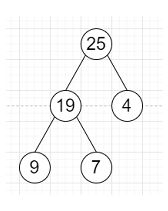# Program to check whether a tree is height balanced or not in C++

Suppose we have a binary tree; we have to check whether its height is balanced or not. We know that for a height balanced tree, for every node in the tree, the absolute difference of the height of its left subtree and the height of its right subtree is 0 or 1.

So, if the input is likethen the output will be True

To solve this, we will follow these steps −

• Define a function dfs(), this will take node,

• if node is null, then −

• return 0

• l := 1 + dfs(left of node)

• r := 1 + dfs(right of node)

• if |l - r| > 1, then −

• ret := false

• return maximum of l and r

• From the main method do the following −

• ret := true

• dfs(root)

• return ret

Let us see the following implementation to get better understanding −

## Example

Live Demo

#include <bits/stdc++.h>
using namespace std;
class TreeNode {
public:
int val;
TreeNode *left;
TreeNode *right;
TreeNode(int x) : val(x), left(NULL), right(NULL) {}
};
class Solution {
public:
bool ret;
int dfs(TreeNode* node){
if(!node)
return 0;
int l = 1 + dfs(node->left);
int r = 1 + dfs(node->right);
if(abs(l - r) > 1)
ret = false;
return max(l, r);
}
bool isBalanced(TreeNode* root) {
ret = true;
dfs(root);
return ret;
}
};
main(){
Solution ob;
TreeNode *root = new TreeNode(25);
root->left = new TreeNode(19);
root->right = new TreeNode(4);
root->left->left = new TreeNode(9);
root->left->right = new TreeNode(7);
cout << (ob.isBalanced(root));
}

## Input

TreeNode *root = new TreeNode(25);
root->left = new TreeNode(19);
root->right = new TreeNode(4);
root->left->left = new TreeNode(9);
root->left->right = new TreeNode(7);

## Output

1